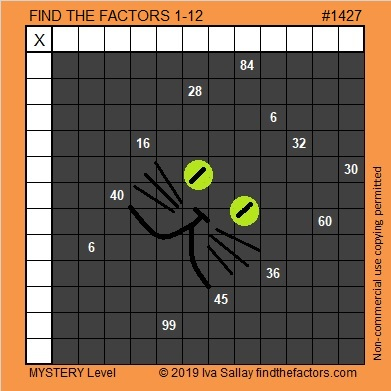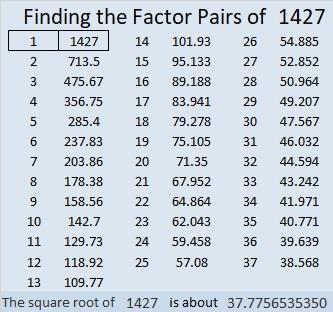# 1427 Mysterious Cat

This mysterious Halloween cat shares twelve clues that can help you solve its puzzle. Each clue is the products of two factors from 1 to 12 multiplied together. Will you be able to solve its mystery?Print the puzzles or type the solution in this excel file: 12 Factors 1419-1429

Now I’ll tell you a little bit about the puzzle number, 1427:

• 1427 is a prime number.
• Prime factorization: 1427 is prime.
• 1427 has no exponents greater than 1 in its prime factorization, so √1427 cannot be simplified.
• The exponent in the prime factorization is 1. Adding one to that exponent we get (1 + 1) = 2. Therefore 1427 has exactly 2 factors.
• The factors of 1427 are outlined with their factor pair partners in the graphic below.

How do we know that 1427 is a prime number? If 1427 were not a prime number, then it would be divisible by at least one prime number less than or equal to √1427. Since 1427 cannot be divided evenly by 2, 3, 5, 7, 11, 13, 17, 19, 23, 29, 31, or 37, we know that 1427 is a prime number.1427 is 272 in BASE 25 because 2(25²) + 7(25) + 2(1) = 1427

This site uses Akismet to reduce spam. Learn how your comment data is processed.